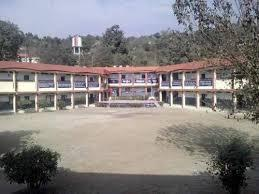# Quiz - 1 Class - 9 Chapter - Number System

15 Questions | Total Attempts: 594Settings• 1.
0.1 can be represented by
• A.

1/10

• B.

1/100

• C.

1/1000

• D.

1/10000

• 2.
Which one of the following is a correct statement?
• A.

Decimal expansion of a rational number is terminating.

• B.

Decimal expansion of a rational number is non-terminating.

• C.

Decimal expansion of an irrational number is terminating.

• D.

Decimal expansion of an irrational number is non- terminating and non- repeating.

• 3.
Which one of the following statements is true?
• A.

The sum of two irrational numbers is always an irrational number.

• B.

The sum of two irrational numbers is always a rational number.

• C.

The sum of two irrational numbers may be a rational number or an irrational number.

• D.

The sum of two irrational numbers is always an integer.

• 4.
Which of the following is a correct statement ?
• A.

Product of two irrational numbers is always irrational.

• B.

Product of a rational and an irrational number is a always irrational.

• C.

Sum of two irrational numbers can never be irrational.

• D.

Sum of an integer and a rational number can never be an integer.

• 5.
Which of these is greatest number?
• A.

0.1

• B.

0.01

• C.

0.001

• D.

0.0001

• 6.
The number 0.318564318564318564.......... is
• A.

A natural number

• B.

An integer

• C.

A rational number

• D.

An irrational number

• 7.
if n is a natural number then, √n is always
• A.

Always a natural number

• B.

Always an irrational number

• C.

Always an irrational number

• D.

Sometimes a natural number and sometimes an irrational number

• 8.
Which of the following numbers can be represented as non-terminating, repeating decimals?
• A.

39/24

• B.

3/16

• C.

3/11

• D.

137/25

• 9.
Every point on a number line represents
• A.

A unique real number

• B.

A natural number

• C.

A rational number

• D.

An irrational number

• 10.
The number 1.272727...... in the form p/q, where p and q are integers and q is not zero, is
• A.

14/9

• B.

14/11

• C.

14/13

• D.

14/15

• 11.
The number 0.333333...... in the form p/q, where p and q are integrals and q is not zero, is
• A.

33/100

• B.

3/10

• C.

1/3

• D.

3/100

• 12.
The smallest rational number by which 1/3 should be multiplied so that its decimal expansion terminates after one place of decimal, is
• A.

1/10

• B.

3/10

• C.

3

• D.

30

• 13.
0.32222222...... when expressed in the form p/q( where p and q are integers and q is not zero)
• A.

8/25

• B.

29/90

• C.

32/99

• D.

32/199

• 14.
Which of the following is irrational ?
• A.

0.15

• B.

0.01516

• C.

0.151615161516.....

• D.

0.50155001500015....

• 15.
The value of 0.232323..... + 0.222222........ is
• A.

0.45454545.....

• B.

0.43434343

• C.

0.45

• D.

0.43

Related TopicsBack to top